Next: Restraints and their derivatives Up: Equations used in the Previous: Equations used in the   Contents   Index

Subsections

## Features and their derivatives

### Distance

Distance is defined by points
and
:
(A.38)

where
(A.39)

The first derivatives of
with respect to Cartesian coordinates are: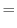(A.40)
(A.41)

### Angle

Angle is defined by points,, and
, and spanned by vectors
and
:
(A.42)

It lies in the interval from 0 to 180
. Internal MODELLER units are radians.

The first derivatives of
with respect to Cartesian coordinates are:
(A.43)
(A.44)
(A.45)

These equations for the derivatives have a numerical instability when the angle goes to 0 or to 180. Presently, the problem is `solved' by testing for the size of the angle; if it is too small, the derivatives are set to 0 in the hope that other restraints will eventually pull the angle towards well behaved regions. Thus, angle restraints of 0 or 180should not be used in the conjugate gradients or molecular dynamics optimizations.

### Dihedral angle

Dihedral angle is defined by points,,, and
(
):
sign
(A.46)

where

 sign
sign
(A.47)

The first derivatives of
with respect to Cartesian coordinates are:
(A.48)

where
(A.49)

and
(A.50)
(A.51)
(A.52)
(A.53)
(A.54)
(A.55)

These equations for the derivatives have a numerical instability when the angle goes to 0. Thus, the following set of equations is used instead [van Schaik et al., 1993]:
(A.56)
(A.57)
(A.58)
(A.59)
(A.60)
(A.61)

The only possible instability in these equations is when the length of the central bond of the dihedral,
, goes to 0. In such a case, which should not happen, the derivatives are set to 0. The expressions for an improper dihedral angle, as opposed to a dihedral or dihedral angle, are the same, except that indicesare permuted to
. In both cases, covalent bonds,
, and
are defining the angle.

### Atomic solvent accessibility

This is the accessibility value calculated by the PSA algorithm (see model.write_data()). This is usually set by the last call to Restraints.make() or Restraints.make_distance(). First derivatives are not calculated, and are always returned as 0.

### Atomic density

Atomic density for a given atom is simply calculated as the number of atoms within a distance energy_data.contact_shell of that atom. First derivatives are not calculated, and are always returned as 0.

### Atomic coordinates

The absolute atomic coordinates
,
and
are available for every point, primarily for use in anchoring points to planes, lines or points. Their first derivatives with respect to Cartesian coordinates are of course simply 0 or 1.Next: Restraints and their derivatives Up: Equations used in the Previous: Equations used in the   Contents   Index
Automatic builds 2013-06-11